## Pre-algebra Learning Tracks for advanced students

Teachers can assign Rocket Math for all their students whether they are behind or advanced. Rocket Math has four pre-algebra topics for those students who already know their multiplication facts. Each of these topics will help them do pre-algebra processes more fluently and to quickly recognize relationships that they have memorized.

## Learning Track 13: Identifying Fractions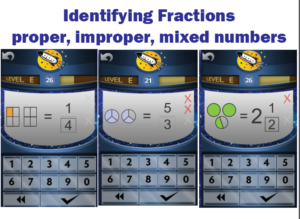When students initially learn about fractions they are often only shown proper fractions. As a result, they have a limited understanding of fractions and can be confused by improper fractions or mixed numbers. Rocket Math prevents this problem.

Students learn to name fractions shown in a picture as parts shaded.  Fractions are always shown with two or more whole units with a number of parts shaded. From the start, we teach students using examples of both proper and improper fractions as well as whole numbers and mixed numbers. Students learn from examples of proper fractions, improper fractions such as 5/3 that are equal to more than 1, and mixed numbers such as 2 and 1/2.

Students learn to identify over 90 different fractions quickly and easily by getting lots of practice. Their understanding of fractions will deepen and become more flexible as they learn to recognize many examples of fractions. Here’s half of the examples students learn from.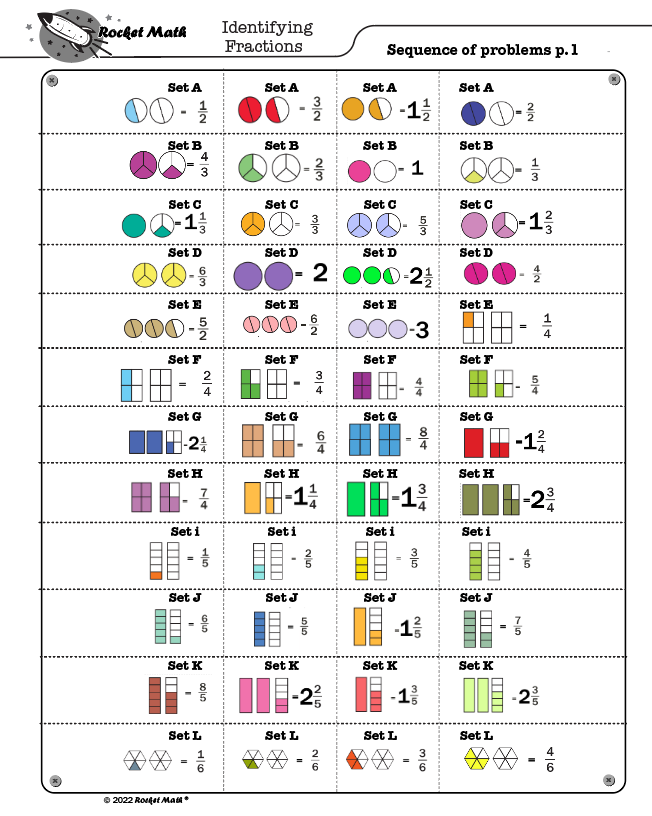## Learning Track 14: Equivalent Fractions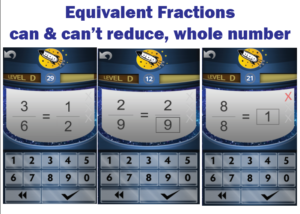Students will memorize the most common equivalent fractions (by reducing them to lowest terms) with this Rocket Math Learning Track. They will also learn to identify a number of fractions, such as 2/9,  that do not “reduce” or for which there are no equivalent fractions in lower terms. Students also learn to recognize a fraction equal to 1 whole in its various forms. When students don’t instantly know the answer they are told the equivalent fraction and given practice on it. The computer gives help in the Online Game.  Their partner gives that help in the Worksheet Program. By the end of the program, students will learn over 90 equivalent fractions. This gives students an excellent start on being able to manipulate fractions quickly and easily.

## Learning Track 15: Factors & Primes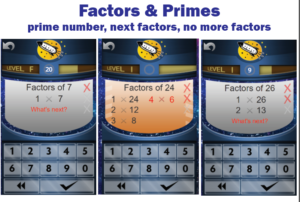Students are required to “find the factors” when dealing with unlike fractions and reducing fractions. Rocket Math Worksheet and Online Game teach students how to find factor pairs. Students learn how to find all the factor pairs and what they all are for many common numbers. They also learn to identify prime numbers and their characteristic of having only one and themselves as factors.

Students learn Dr. Don’s foolproof method for finding the factor pairs in order. https://www.rocketmath.com/2020/12/29/foolproof-method-finding-factor-pairs/ By this method they know the “last” factor pair when they see it. When the game asks “What’s next?” students can provide the next pair of factors or click the checkmark to indicate there are no more factors. When students go through this Learning Track they will no longer hesitate when asked for the factors of common numbers.

## Learning Track 16: Fraction & Decimal Equivalents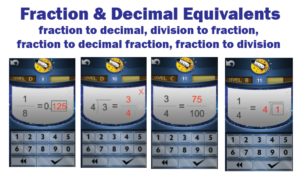Learning common fractions and their decimal equivalents, learning fractions and their fraction equivalents in 10ths or 100ths.

Common fraction and decimal equivalents should not require a laborious process to “figure out.” Students should just know these, so this Learning Track in the Online Game allows them to memorize a bunch of common decimal and fraction equivalents.

Having a facility with a lot of fraction and decimal equivalents means faster computation as well as a way to check their process when manipulating fractions and decimals. Students also learn another essential pre-algebra skill that often confuses them.  They learn to correctly and fluently translate a fraction into a division problem and vice-versa.

“Test Drive” any of the 16 Learning Tracks in our Online Game demo accounts.